# Seminar 4

In this seminar, we will use Y.RData file that we created in the Seminar 2. We focus on coding GARCH model using rugarch package. Please review the theory of GARCH that we discussed in the lecture.

The plan for this week:

The following sections and subsections are materials for students to learn by themselves: Other GARCH specifications, GARCH Stationarity and Half-life

We first load the packages for this seminar:

In :
library(rugarch)
library(tseries)


## Univariate GARCH models¶

In this class we will work with the GARCH family, which was originally proposed in 1982 by Robert Engle. Most volatility models used in industry nowadays derive from this formulation.

Returns have a conditional distribution, which we will initially assume to be normal:

$$Y_{t} \sim \mathcal{N}\left(0,\sigma_t^2\right)$$

Which can be written as: $Y_t = \sigma_{t}Z_{t}$, where $Z_{t} \sim \mathcal{N}\left(0,1\right)$. $Z_t$ is called residual.

The $\textrm{GARCH}\left(L_1,L_2\right)$ model is:

$$\sigma_t^2 = \omega + \sum_{i=1}^{L_1}\alpha_{i}Y^2_{t-i} + \sum_{j=1}^{L_2}\beta_{j}\sigma^2_{t-j}$$

Where:
$\alpha$ is news.
$\beta$ is memory.
The size of $\left(\alpha + \beta\right)$ determines how quickly the predictability of the process dies out.

We are going to use the J.P. Morgan returns from our Y.RData file to run various GARCH type models discussed in Chapter 2.

In :
# Load the data

# Save the returns in a variable called y
y <- Y$JPM  In : # Simple plot plot(y, type = "l", main = "Returns for JP Morgan")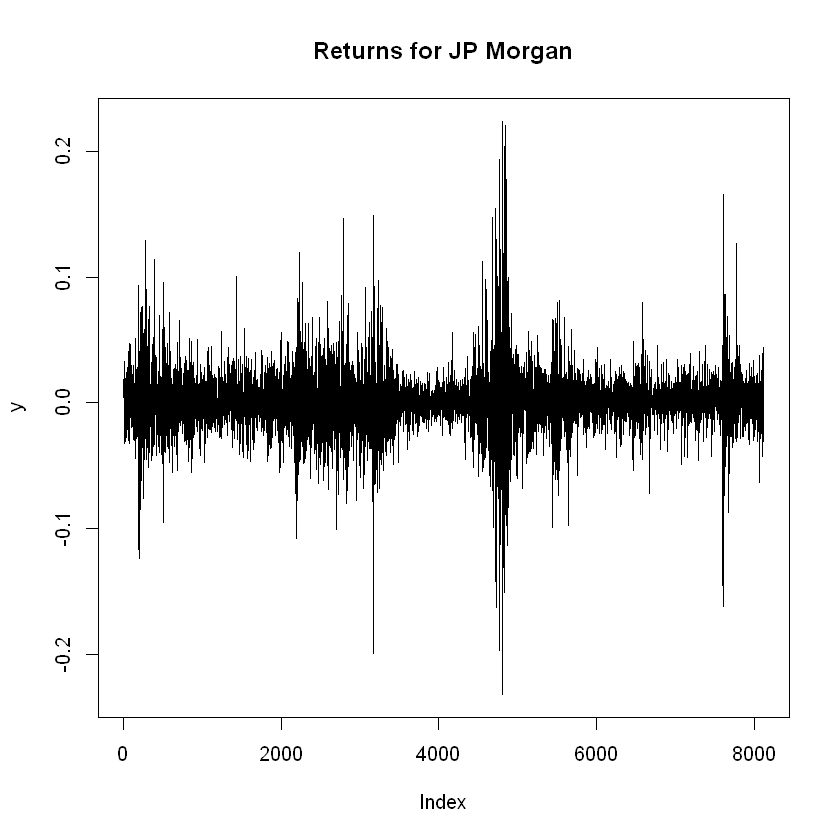In : # Autocorrelations acf(y, main = "Autocorrelations of JPM returns") acf(y^2, main = "Autocorrelations of JPM returns squared")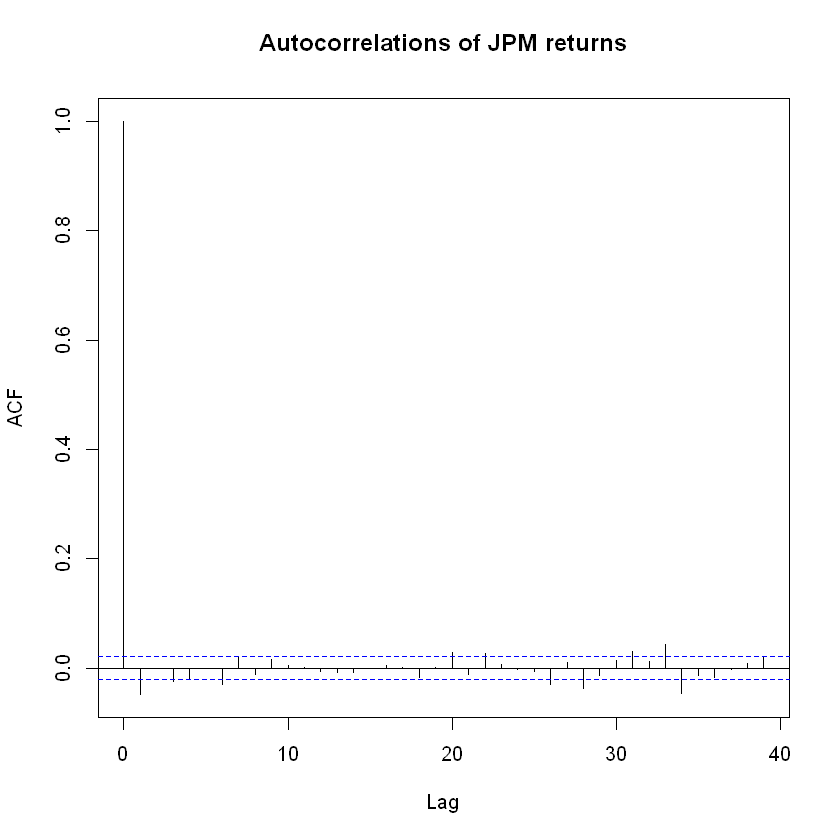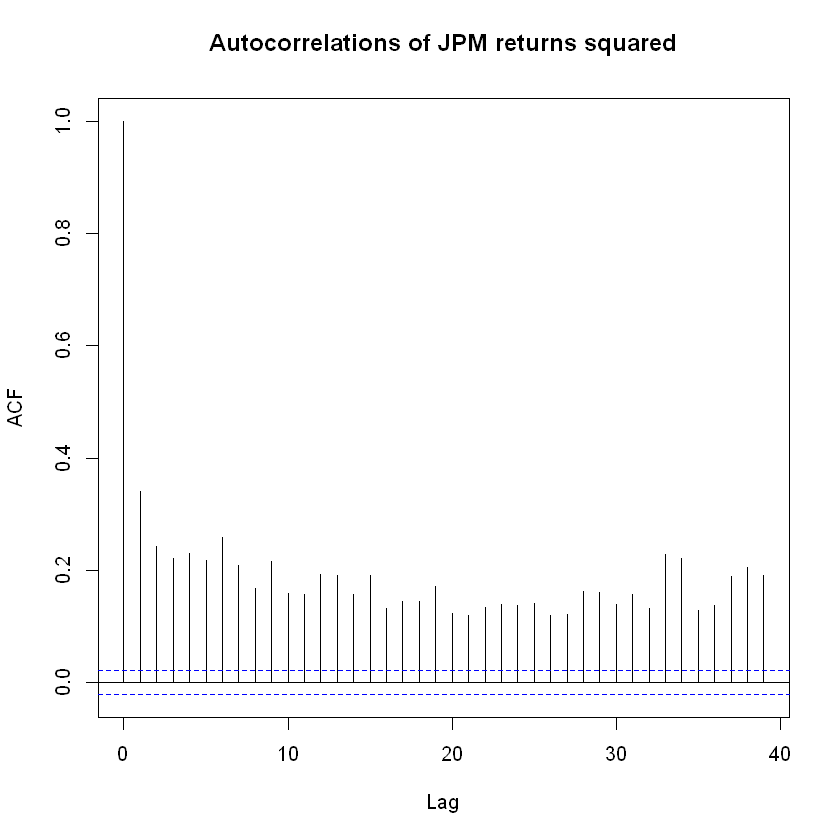The autocorrelation of the returns squared are significant for every lag, meaning that we see a behavior that can be modeled with GARCH. We will use the rugarch library for building univariate GARCH models. The documentation for it can be found here: https://cran.r-project.org/web/packages/rugarch/rugarch.pdf To build a univariate GARCH model, we first specify the type of GARCH model. ### GARCH model specification¶ We can specify the type of GARCH model we want to fit using the ugarchspec() function. For example, we can specify the number of lags, if the model includes leverage or power effects, whether the mean should be included or not, and the conditional distribution of the returns. The common syntax we will use inside ugarchspec() is: Type of model: variance.model = list(model = "type of model"), Whether to include or not a mean: mean.model = list(armaOrder = c(0,0), include.mean = FALSE), Type of conditiona distribution: distribution.model = "std" To get a detailed explanation on how to use all the options of ugarchspec(), you can type ?ugarchspec in the console. ### Default GARCH¶ First we will run the default version, which is a conditionally normal GARCH(1,1) model with non-zero mean which follows an ARMA(1,1) process: In : # Create the specifications default_spec <- ugarchspec()  In : # We can call the variable to see what's inside default_spec  *---------------------------------* * GARCH Model Spec * *---------------------------------* Conditional Variance Dynamics ------------------------------------ GARCH Model : sGARCH(1,1) Variance Targeting : FALSE Conditional Mean Dynamics ------------------------------------ Mean Model : ARFIMA(1,0,1) Include Mean : TRUE GARCH-in-Mean : FALSE Conditional Distribution ------------------------------------ Distribution : norm Includes Skew : FALSE Includes Shape : FALSE Includes Lambda : FALSE  Once we have specified the model, we can fit our data into it using ugarchfit(): In : # Fit the model to the data using ugarchfit default_garch <- ugarchfit(spec = default_spec, data = y)  In : # Call the variable default_garch  *---------------------------------* * GARCH Model Fit * *---------------------------------* Conditional Variance Dynamics ----------------------------------- GARCH Model : sGARCH(1,1) Mean Model : ARFIMA(1,0,1) Distribution : norm Optimal Parameters ------------------------------------ Estimate Std. Error t value Pr(>|t|) mu 0.000819 0.000171 4.80241 0.000002 ar1 0.176238 0.455092 0.38726 0.698566 ma1 -0.166105 0.455878 -0.36436 0.715587 omega 0.000003 0.000002 2.06352 0.039063 alpha1 0.077755 0.010591 7.34146 0.000000 beta1 0.917528 0.011261 81.48134 0.000000 Robust Standard Errors: Estimate Std. Error t value Pr(>|t|) mu 0.000819 0.000175 4.68018 0.000003 ar1 0.176238 0.187413 0.94037 0.347027 ma1 -0.166105 0.188466 -0.88135 0.378127 omega 0.000003 0.000009 0.35602 0.721822 alpha1 0.077755 0.057656 1.34860 0.177465 beta1 0.917528 0.062852 14.59829 0.000000 LogLikelihood : 21011.23 Information Criteria ------------------------------------ Akaike -5.1699 Bayes -5.1647 Shibata -5.1699 Hannan-Quinn -5.1681 Weighted Ljung-Box Test on Standardized Residuals ------------------------------------ statistic p-value Lag 0.2763 0.5991 Lag[2*(p+q)+(p+q)-1] 1.6479 0.9940 Lag[4*(p+q)+(p+q)-1] 3.4515 0.8111 d.o.f=2 H0 : No serial correlation Weighted Ljung-Box Test on Standardized Squared Residuals ------------------------------------ statistic p-value Lag 6.654 0.009894 Lag[2*(p+q)+(p+q)-1] 9.976 0.009338 Lag[4*(p+q)+(p+q)-1] 11.774 0.020413 d.o.f=2 Weighted ARCH LM Tests ------------------------------------ Statistic Shape Scale P-Value ARCH Lag 0.5911 0.500 2.000 0.4420 ARCH Lag 0.8980 1.440 1.667 0.7634 ARCH Lag 2.3007 2.315 1.543 0.6538 Nyblom stability test ------------------------------------ Joint Statistic: 23.6911 Individual Statistics: mu 0.05573 ar1 1.20987 ma1 1.22022 omega 3.19859 alpha1 0.27334 beta1 0.46699 Asymptotic Critical Values (10% 5% 1%) Joint Statistic: 1.49 1.68 2.12 Individual Statistic: 0.35 0.47 0.75 Sign Bias Test ------------------------------------ t-value prob sig Sign Bias 1.1738 2.405e-01 Negative Sign Bias 4.5956 4.380e-06 *** Positive Sign Bias 0.8033 4.219e-01 Joint Effect 24.7751 1.721e-05 *** Adjusted Pearson Goodness-of-Fit Test: ------------------------------------ group statistic p-value(g-1) 1 20 177.2 1.078e-27 2 30 214.0 4.198e-30 3 40 239.0 1.447e-30 4 50 258.5 3.002e-30 Elapsed time : 0.7248468  The function provides comprehensive information on the model fit. It includes the optimal parameters estimates, standard errors, tests for significance, likelihood, information criteria, and several other tests. Since this is too much information in the same place, we can tell R what particular information we are interested in. By using the @ symbol we can extract the contents from an object. For example, by running: In : # Check the content of default_garch names(default_garch@fit)  1. 'hessian' 2. 'cvar' 3. 'var' 4. 'sigma' 5. 'condH' 6. 'z' 7. 'LLH' 8. 'log.likelihoods' 9. 'residuals' 10. 'coef' 11. 'robust.cvar' 12. 'A' 13. 'B' 14. 'scores' 15. 'se.coef' 16. 'tval' 17. 'matcoef' 18. 'robust.se.coef' 19. 'robust.tval' 20. 'robust.matcoef' 21. 'fitted.values' 22. 'convergence' 23. 'kappa' 24. 'persistence' 25. 'timer' 26. 'ipars' 27. 'solver' We see all the content provided by the ugarchfit() function. We can access any element of this list by using the $ sign:

In :
# Extract a particular element
default_garch@fit$coef  mu 0.000819100731887893 ar1 0.176237519261007 ma1 -0.166105161822789 omega 3.17179088199319e-06 alpha1 0.0777550630575975 beta1 0.917528083558844 Another way of accessing this information is: In : # Extracting coefficients with coef() coef(default_garch)  mu 0.000819100731887893 ar1 0.176237519261007 ma1 -0.166105161822789 omega 3.17179088199319e-06 alpha1 0.0777550630575975 beta1 0.917528083558844 Let's say we are particularly interesting in$\omega$. We can access it by: In : # Accessing omega using its index coef(default_garch)  omega: 3.17179088199319e-06 However, using the name instead of the index can prevent potential mistakes in our code (for example, if the package is updated and the list reordered): In : # Accessing omega using its name coef(default_garch)["omega"]  omega: 3.17179088199319e-06 We can view the likelihood of the fitted model with: In : likelihood(default_garch)  21011.2265809712 ## Plotting the GARCH output¶ A very useful feature of rugarch package is plotting. If we call the function plot() on a fitted GARCH model like default_garch, the following interactive menu will appear: Make a plot selection (or 0 to exit): 1. Series with 2 Conditional SD Superimposed 2. Series with 1% VaR Limits 3. Conditional SD (vs |returns|) 4. ACF of Observations 5. ACF of Squared Observations 6. ACF of Absolute Observations 7. Cross Correlation 8. Empirical Density of Standardized Residuals 9. QQ-Plot of Standardized Residuals 10. ACF of Standardized Residuals 11. ACF of Squared Standardized Residuals 12. News-Impact Curve  We can visualize any of the plots by selecting the corresponding number. The plots for the default_garch are (The plot is different for different time span): 123456789101112These plots provide us with plenty of information to analyze the fit and results of our model. For example, from Plot 8 and Plot 9 we can see that the distribution of residuals in our model does not correctly fit under a normal distribution. As expected, there is an excess of observations around the mean in the histogram in Plot 8, and in Plot 9 we see the presence of fat tails in our observations. A key output of the GARCH model is the estimated conditional volatities, the sequence$\sigma_t$. This sequence is used for generating the first three plots shown above. We can access the sequence through default_garch@fit$sigma.

In :
# Plotting the estimated conditional volatility
lines(non_stationary_GARCH@fit$sigma, col="red") legend("topleft", legend = c("Stationary", "Non-stationary"), lty = 1, col = c("black", "red"))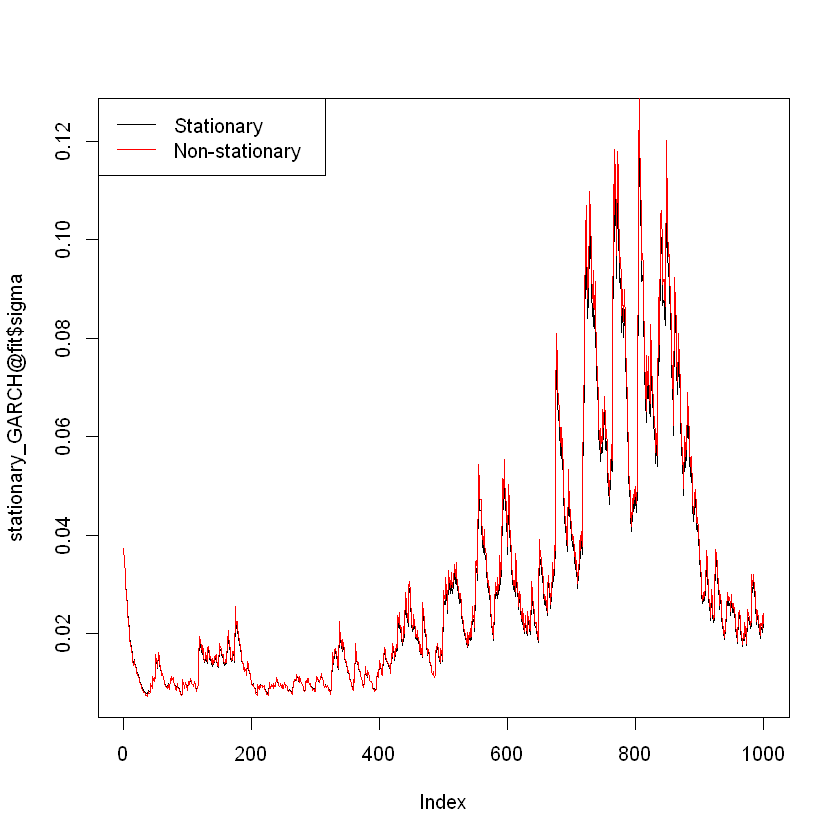However, they are not exactly the same: In : # Create a new variable with the absolute difference between stationary and non-stationary dif <- abs(stationary_GARCH@fit$sigma - non_stationary_GARCH@fit$sigma) # Plot it plot(dif, type = "l", main = "Distance in Stationary and Non-stationary models cond volatility")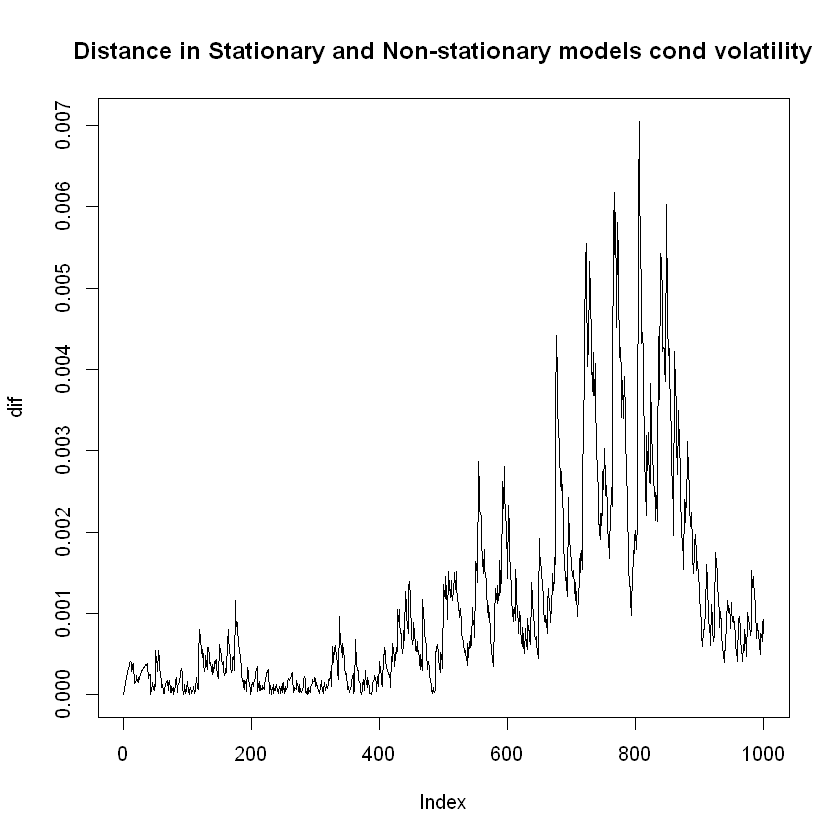The distance between the estimated conditional volatility of the stationary and non-stationary model resembles the shape of the estimated conditional volatility. ## Assess Models and Testing¶ ### Summary of our models¶ The following table summarizes the coefficients for the models we have built: ARCH GARCH tGARCH apARCH tapARCH$\omega$0.00031 2.20394e-06 2.61938e-06 6.59773-05 7.09693e-05$\alpha$0.49309 0.06364 0.07995 0.07320 0.08147$\beta$- 0.93288 0.91809 0.93071 0.92659$\nu$- - 6.10894 - 6.48193$\gamma$- - - 0.47001 0.48501$\delta$- - - 1.29540 1.25297 ### Likelihood ratio test¶ Consider two models, where one is nested inside the other. This can be the case of the$\textrm{ARCH(1)}$, which is a subset of a$\textrm{GARCH(1,1)}$. We can call the nested model restricted (R), and the other unrestricted (U). By definition: $$\log \mathcal{L}_R \leq \log \mathcal{L}_U$$ Two times the difference between the unrestricted and restricted likelihood is distributed as a$\chi^2$with degrees of freedom equal to the number of restrictions: $$\textrm{LR} = 2\left(\log \mathcal{L}_U - \log \mathcal{L}_R\right) \sim \chi^2_{\left(\textrm{number of restrictions}\right)}$$ The number of restrictions means how many parameters we are excluding in the restricted model. For example, if we consider the ARCH vs GARCH models: In : # Number of restrictions ARCH vs GARCH coef(ARCH) coef(GARCH)  omega 0.000307197426429236 alpha1 0.493088202795947 omega 3.12318841360853e-06 alpha1 0.0762591600110328 beta1 0.919048703948558 The ARCH model, nested inside the GARCH model, has one parameter less. So in this case the number of restrictions which serves as the degrees of freedom in the chi-square distribution, would be one. In the case of the ARCH vs the tGARCH model: In : # Number of restrictions ARCH vs tGARCH coef(ARCH) coef(tGARCH)  omega 0.000307197426429236 alpha1 0.493088202795947 omega 2.61938388312584e-06 alpha1 0.0799500636254105 beta1 0.918090748191427 shape 6.10893848251779 The ARCH model has two parameters while the tGARCH has four, so we would be dealing with two degrees of freedom. We can easily implement the LR test using the likelihood output from the ugarchfit() function. Let's perform the test for the ARCH(1) and GARCH(1,1) models we created: In : # Creating the LR statistic - using cat() for output display cat(" Likelihood of ARCH: ", round(likelihood(ARCH),2), "\n", "Likelihood of GARCH: ", round(likelihood(GARCH),2), "\n", "2 * (log Lu - log Lr): ", round(2*(likelihood(GARCH)-likelihood(ARCH)),2))   Likelihood of ARCH: 19803.9 Likelihood of GARCH: 20999.02 2 * (log Lu - log Lr): 2390.24 The value of the test statistic is 2341. How do we know if this is above the critical value for a Chi-square with one degree of freedom and a confidence level of 95%? We can use the qchisq() function to find the 95% quantile of the Chi-square. In : # 95% quantile of chi-square qchisq(p = 0.95, df = 1)  3.84145882069412 The critical value is 3.84, which is smaller than 2341. This means we have enough evidence to reject that the two models perform similarly. We can also use pchisq() to find the p-value of the test statistic: In : # Adding the p-value p.value <- 1 - pchisq(2*(likelihood(GARCH)-likelihood(ARCH)), df = 1) cat(" Likelihood of ARCH: ", round(likelihood(ARCH),2), "\n", "Likelihood of GARCH: ", round(likelihood(GARCH),2), "\n", "2 * (Lu - Lr): ", round(2*(likelihood(GARCH)-likelihood(ARCH)),2), "\n", "p-value:", p.value)   Likelihood of ARCH: 19803.9 Likelihood of GARCH: 20999.02 2 * (Lu - Lr): 2390.24 p-value: 0 We can create a Test() function that takes two fitted models and gives an output with the likelihoods and p-values: In : # Creating a function for Likelihood Tests Test <- function(restricted, unrestricted) { # Specifying the degrees of freedom as the number of restrictions df <- length(unrestricted@fit$coef) - length(restricted@fit$coef) # Creating the statistic lr <- 2*(likelihood(unrestricted) - likelihood(restricted)) # Finding its p-value p.value <- 1 - pchisq(lr, df) # Output cat("Degrees of freedom:", df, "\n", "Likelihood of unrestricted model:", likelihood(unrestricted), "\n", "Likelihood of restricted model:", likelihood(restricted), "\n", "LR: 2*(Lu-Lr):", lr, "\n", "p-value:", p.value ) }  Using our new Test() function, let's test the GARCH and tapARCH models: In : # Testing the GARCH and tapARCH Test(GARCH, tapARCH)  Degrees of freedom: 3 Likelihood of unrestricted model: 21302.43 Likelihood of restricted model: 20999.02 LR: 2*(Lu-Lr): 606.8187 p-value: 0 ### Residual Analysis¶ We have assumed returns to be conditionally normally distributed. We can test this with a Jarque-Bera test for normality and a Ljung-Box test for autocorrelation of returns and returns-squared, and do a QQ plot to see the fit. Let's perform this test with the output of our GARCH(1,1) model: In : # Performing residual analysis residuals <- GARCH@fit$residuals

In :
# Autocorrelation of residuals
acf(residuals, main = "Autocorrelation of residuals")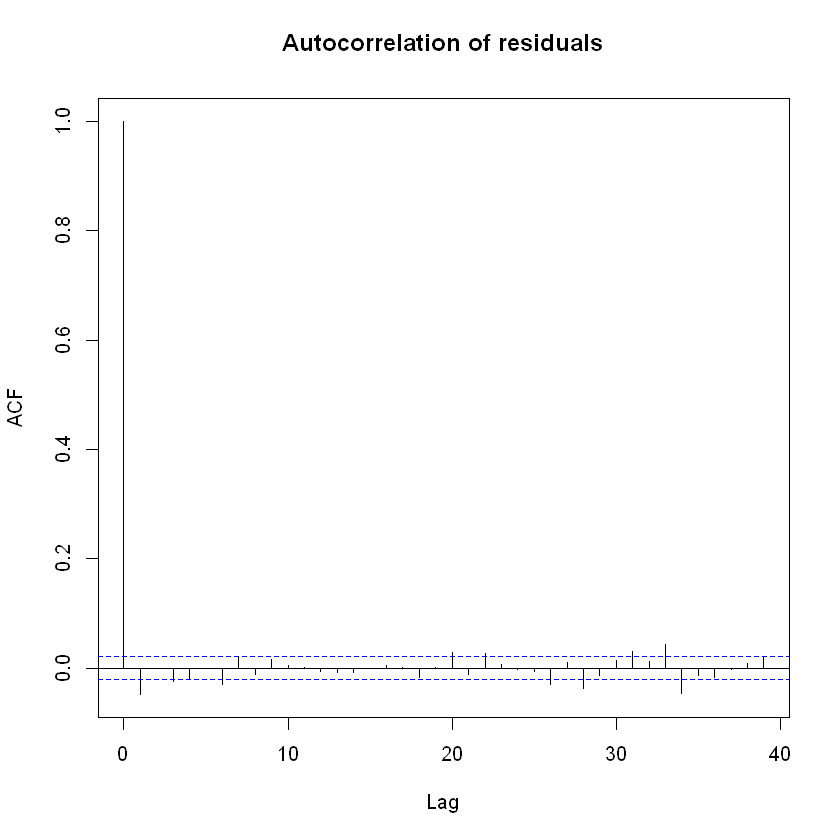In :
# Autocorrelation of residuals squared
acf(residuals^2, main = "Autocorrelation of residuals squared")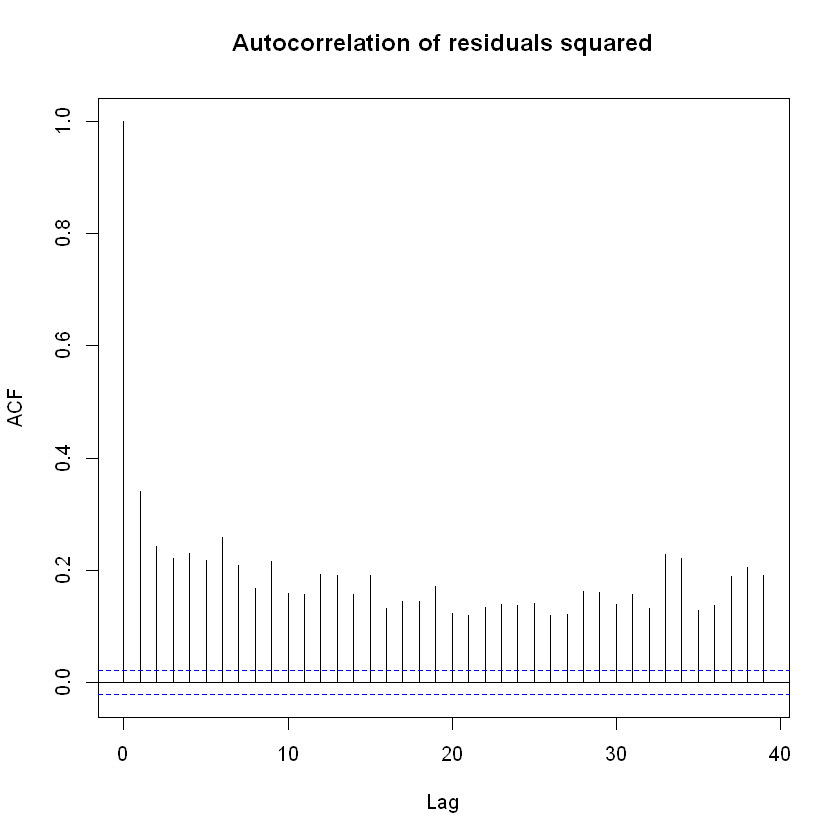In :
# Jarque-Bera test for Normality
jarque.bera.test(residuals)

	Jarque Bera Test

data:  residuals
X-squared = 45517, df = 2, p-value < 2.2e-16

In :
# Ljung-Box test for residuals
Box.test(residuals, type = "Ljung-Box")

	Box-Ljung test

data:  residuals
X-squared = 19.221, df = 1, p-value = 1.164e-05

In :
# Ljung-Box test for residuals squared
Box.test(residuals^2, type = "Ljung-Box")

	Box-Ljung test

data:  residuals^2
X-squared = 936.97, df = 1, p-value < 2.2e-16

In :
# qqPlot for residuals under a normal distribution
qqPlot(residuals)

Error in qqPlot(residuals): could not find function "qqPlot"
Traceback:

In :
# qqPlot for residuals under a Student-t with 3 degrees of freedom
qqPlot(residuals, distribution = "t", df = 3, envelope = FALSE)

Error in qqPlot(residuals, distribution = "t", df = 3, envelope = FALSE): could not find function "qqPlot"
Traceback:


## Half-life and simulations¶

### Half-life (Not covered in class)¶

We are interested in how quickly shocks die out to understand the memory present in the data. The half-life is defined as the number of periods, $n^*$, it takes for conditional variance to revert back halfway towards unconfitional variance: $$\sigma^2_{t+n^*,t} - \sigma^2 = \frac{1}{2}\left(\sigma^2_{t+1,t}-\sigma^2\right)$$
$$\left(\alpha + \beta \right)^{n^*-1} \left(\sigma^2_{t+1,t}-\sigma^2\right) = \frac{1}{2}\left(\sigma^2_{t+1,t}-\sigma^2\right)$$
$$n^* = 1 + \frac{\log\left(\frac{1}{2}\right)}{\log\left(\alpha + \beta \right)}$$

So, as $\left(\alpha + \beta \right) \rightarrow 1$, $n^* \rightarrow \infty$, the memory is infinite. We can easily calculate the half-life from our model:

In :
# Half-life
# We use unname() to remove the names of the objects
alpha <- unname(coef(GARCH)['alpha1'])
beta <- unname(coef(GARCH)['beta1'])
half_life <- 1 + (log(0.5)/log(alpha+beta))
cat("The Half-life for our GARCH(1,1) is", half_life, "time periods.")

The Half-life for our GARCH(1,1) is 148.3784 time periods.

### Simulations¶

The package rugarch allows us to use the function ugarchsim() to simulate series using the fitted model. Since simulations involve the use of random numbers, every simulation will be different from each other (unless we specify seeds for replicability).

As a exercise, we can do 1000 simulations using our GARCH(1,1) model and use the matplot() function to see how our simulated returns and volatility would look like:

In :
# Create 1000 simulations from our fitted model
simulations <- ugarchsim(GARCH, m.sim = 1000)

# Plot the simulated returns
matplot(simulations@simulation$seriesSim, type = "l", main = "Simulations of returns")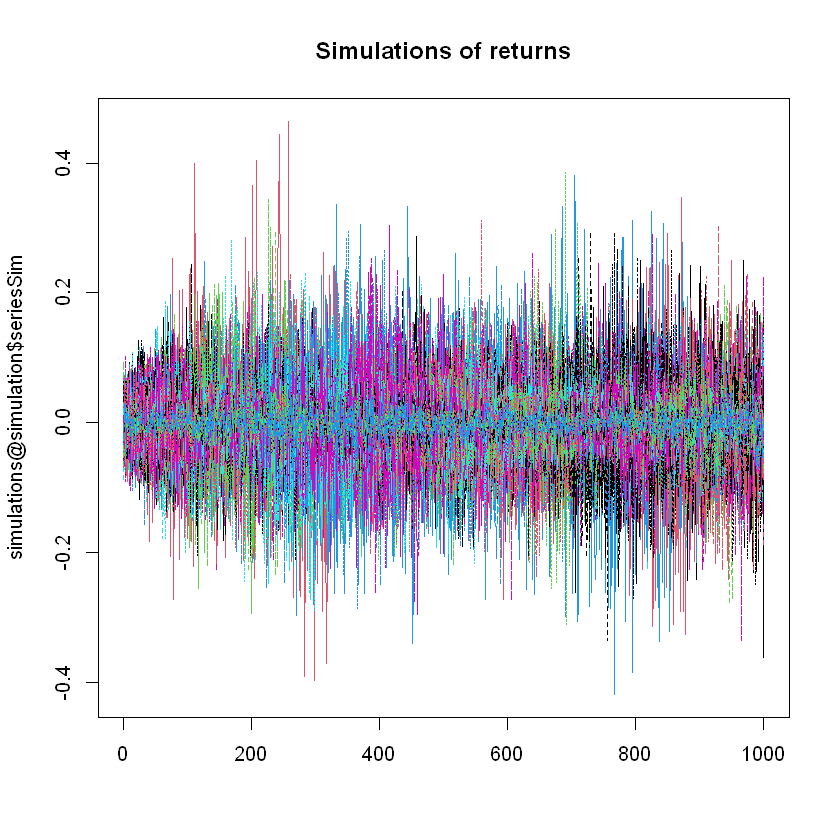In : # Plot the simulated volatilities matplot(simulations@simulation$sigmaSim, type = "l",
main = "Simulations of volatility")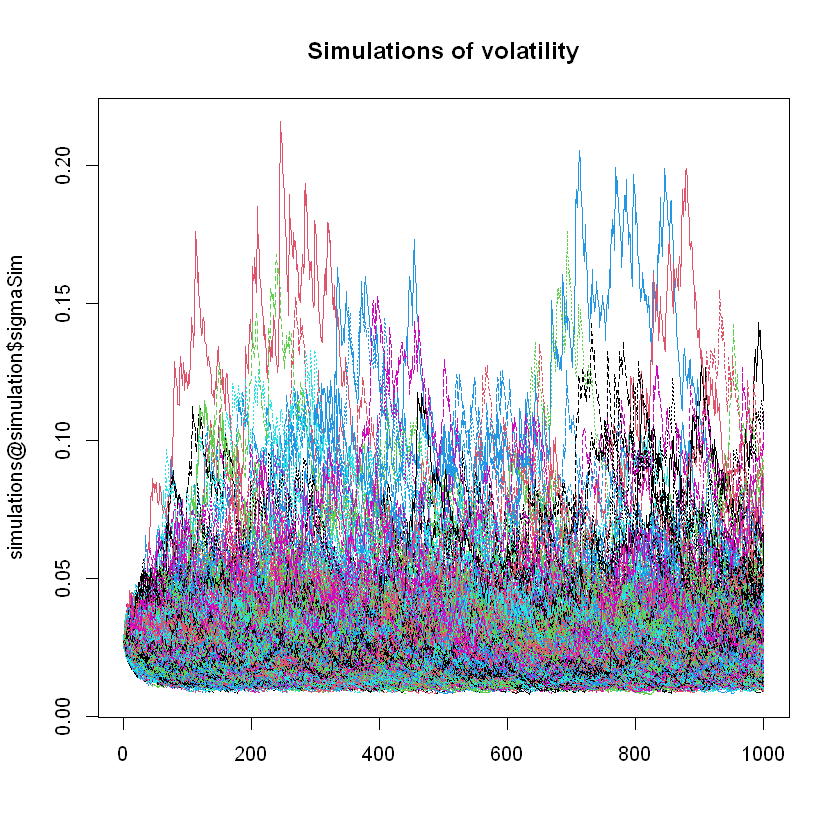### Monte-Carlo simulation¶

We can take advantage of ugarchsim() to do a quick Monte Carlo study. We know the coefficients of our model are:

In :
coef(GARCH)

omega
3.12318841360853e-06
alpha1
0.0762591600110328
beta1
0.919048703948558

We can fit a GARCH model to every simulation of returns, store the coefficients, and see how they are distributed and the relationship to the true coefficients. The law of large numbers tells us that for a large number of simulations, the distribution should converge to the true values:

In :
# Number of simulations
sim <- 1000

# Create vectors to hold the fitted parameters
omega_sim <- vector(length = sim)
alpha_sim <- vector(length = sim)
beta_sim <- vector(length = sim)

# Specify the GARCH we will use
spec <- ugarchspec(
variance.model = list(garchOrder = c(1,1)),
mean.model = list(armaOrder = c(0,0), include.mean = FALSE)
)

# Create the simulations
simulations <- ugarchsim(GARCH, m.sim = sim)

# Fit a GARCH for every simulation and store the parameter values
for (i in 1:sim) {
# Fit the GARCH model
temp <- ugarchfit(spec = spec1, data = simulations@simulation\$seriesSim[,i])
# Add parameters to vectors created
omega_sim[i] <- unname(coef(temp)['omega'])
alpha_sim[i] <- unname(coef(temp)['alpha1'])
beta_sim[i] <- unname(coef(temp)['beta1'])
}

In :
# Omega

# Distribution
hist(omega_sim, col = "lightgray", main = "Distribution of simulated omega")

# Adding a vertical line on the true parameter
abline(v = coef(GARCH)['omega'], col = "red", lwd = 2)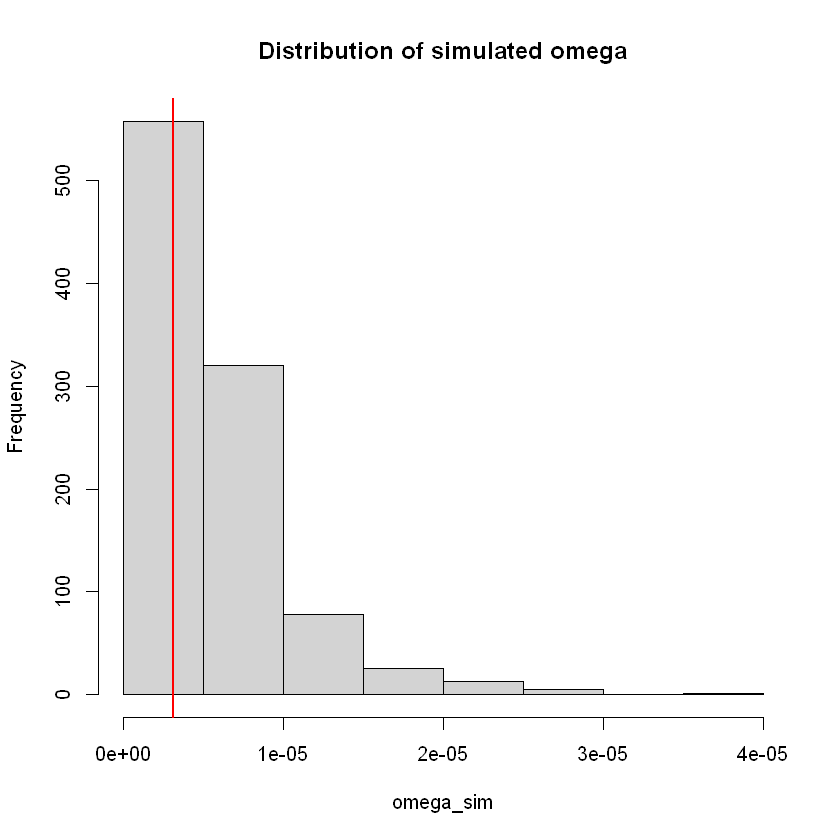In :
# Alpha

# Distribution
hist(alpha_sim, col = "lightgray", main = "Distribution of simulated alpha")

# Adding a vertical line on the true parameter
abline(v = coef(GARCH)['alpha1'], col = "red", lwd = 2)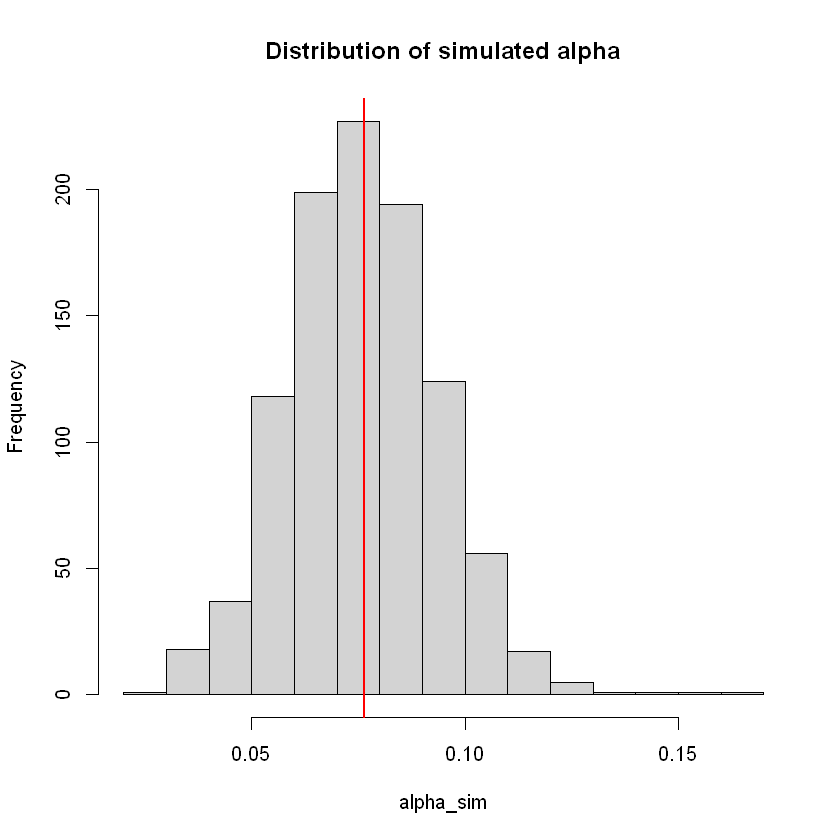In :
# Beta

# Distribution
hist(beta_sim, col = "lightgray", main = "Distribution of simulated beta")

# Adding a vertical line on the true parameter
abline(v = coef(GARCH)['beta1'], col = "red", lwd = 2)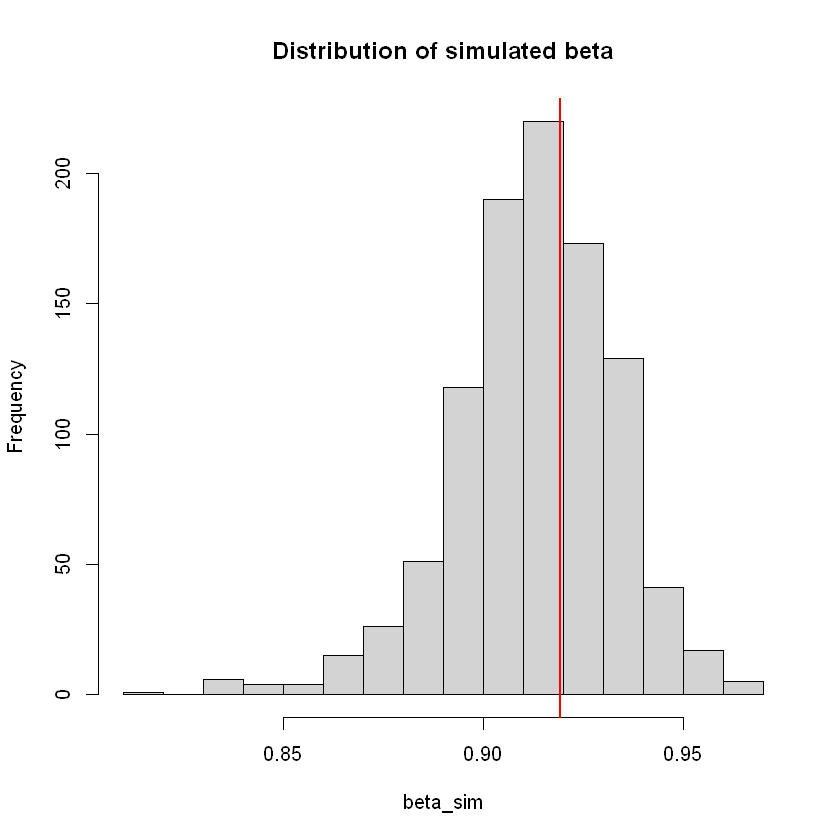## Recap¶

In this seminar we have covered:

• Building univariate GARCH models using R
• Accessing the output of a fitted GARCH model
• Plotting GARCH output
• Fitting ARCH, tARCH, apARCH and tapARCH models
• Performing Likelihood ratio tests
• Creating a function
• Doing residual analysis
• Half-life
• Simulations with fitted GARCH models
• Monte Carlo experiment for GARCH
• Variance stationarity

Some new functions used:

• ugarchspec()
• ugarchfit()
• ugarchsim()
• coef()
• likelihood()
• cat()
• function()

For more discussion on the material covered in this seminar, refer to Chapter 2: Univariate volatility modeling on Financial Risk Forecasting by Jon Danielsson.

Acknowledgements: Thanks to Alvaro Aguirre and Yuyang Lin for creating these notebooks